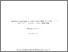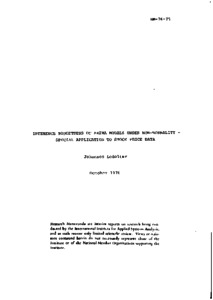Inference Robustness of ARIMA Models under Non-normality - Special Application to Stock Price Data

Ledolter, J. (1976). Inference Robustness of ARIMA Models under Non-normality - Special Application to Stock Price Data. IIASA Research Memorandum. IIASA, Laxenburg, Austria: RM-76-075Preview
Text
RM-76-075.pdf

Abstract

Wold's decomposition theorem states that every weakly stationary stochastic process can be decomposed into orthogonal shocks. For practical reasons, however, it is desirable to employ models which use parameters parsimoniously. Box and Jenkins show how parsimony can be achieved by representing the linear process in terms of a small number of autoregressive and moving average terns (ARIMA-models). The Gaussian hypothesis assumes that the shocks follow a normal distribution with fixed mean and variance. In this case the process is characterized by first and second order moments. The normality assumption seems to be reasonable for many kinds of series. However, it was pointed out by Kendall, Mandelbrot, and Fama that particularly for stock price data the distribution of the shocks appears leptokurtic.

In this paper we investigate the sensitivity of ARIMA models to non-normality of the distribution of the shocks. We suppose that the distribution function of the shocks is a member of the symmetric exponential power family, which includes the normal as well as leptokurtic and platikurtic distributions. A Bayesian approach is adopted and the inference robustness of ARIMA models with respect to (i) the estimation of parameters, and (ii) the forecasts of future observations is discussed.

Item Type: Monograph (IIASA Research Memorandum) System and Decision Sciences - Core (SDS) IIASA Import 15 Jan 2016 01:43 27 Aug 2021 17:08 http://pure.iiasa.ac.at/609View Item